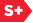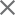### Proceedings Paper

A class of heavy-tailed multivariate non-Gaussian probability models for wavelet coefficients
Format Member Price Non-Member Price
PDF \$17.00 \$21.00

Paper Abstract

It is well documented that the statistical distribution of wavelet coefficients for natural images is non-Gaussian and that neighboring coefficients are highly dependent. In this paper, we propose a new multivariate non-Gaussian probability model to capture the dependencies among neighboring wavelet coefficients in the same scale. The model can be expressed as K exp(-||w||) where w is a N-element vector of wavelet coefficients and ||w|| is a convex combination of l2 norms over subspaces of RN. This model includes the commonly used independent Laplacian model as a special case but it has many more degrees of freedom. Based on this model, the corresponding non-linear threshold (shrinkage) function for denoising is derived using Bayesian estimation theory. Although this function does not have a closed-form solution, a successive substitution method can be used to numerically compute it.

Paper Details

Date Published: 13 November 2003
PDF: 8 pages
Proc. SPIE 5207, Wavelets: Applications in Signal and Image Processing X, (13 November 2003); doi: 10.1117/12.504644
Show Author Affiliations
Fei Shi, Polytechnic Univ. (United States)
Ivan W. Selesnick, Polytechnic Univ. (United States)

Published in SPIE Proceedings Vol. 5207:
Wavelets: Applications in Signal and Image Processing X
Michael A. Unser; Akram Aldroubi; Andrew F. Laine, Editor(s)